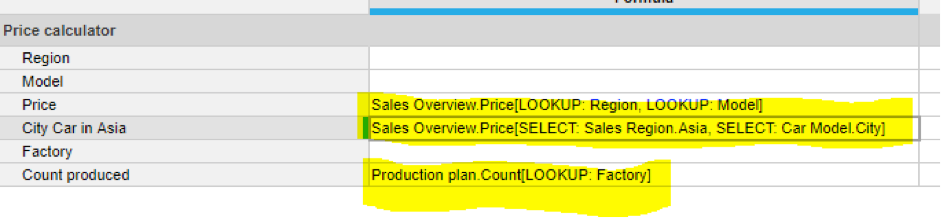Search
• Bedford Coach

# The Bedford Coach - Key Formulas - SUM vs LOOKUP vs SELECTFor new Anaplan model builders; SUM, LOOKUP & SELECT functions can often become confusing! In this case I will provide an overview of cases when each function should be used.

Anaplan definition:

• LOOKUP: The function looks up a number, Boolean, time period, list item, text, or date value in a list or a time period from a source module using one or more common mappings.

• SUM: Sum values based on a list-formatted or time period-formatted line item or property in the source that matches a dimension in the target. Each mapping is a line item or property with data type specified to be list values from one of the dimensions of the result line item. SUM works by using the mappings you enter for the function parameters as a set of determining criteria for the values in the source to be summed in the result.

• SELECT: The SELECT function is used to identify a list item to use from one or more hierarchy lists to filter the source module data. This function works in conjunction with the other dimensions in the module to return dependent values.

The BasicsSELECT: x[SELECT: y] where:

X: Source: Number, Boolean, date, time period, list, text

Y: Time period, list

LOOKUP: x[LOOKUP: y] where:

X: Source: Number, Boolean, date, time period, list, text

Y: Mapping: List, time period

Note: This function requires one and accepts multiple arguments as mappings—each argument must be separated by a comma.

SUM: x[SUM: y] where:

X: Column holding the values to SUM

Y: Columns to use as cross references. Multiple cross references are allowed. x[SUM: y, SUM: z, ...])

Typical use cases

Here is a basic module by region and model, with count, price, revenue & factory as line items:List to List

Here is a direct link to a line item. This is because Sales Region is a list in the module below and is also a list in the reference module Sales Overview above.SUM

If you want to link a list to a line item, we would need to use the SUM formula. This will SUM the production plan by Factory.LOOKUP & SELECT

Here we are looking at line items in the current modules and lists in the reference module.

LOOKUP: In this example LOOKUP works by looking up a line item in the same module you are working on; as shown below.

SELECT: Here you need to specify a list item you want to select, so in the case below where we have selected City Bike in Asia – we have specifically chose Sales region to be ‘Asia’ and the model range to be ‘City’.Linking Line item to Line item

It’s recommended you use a module where you have factory as a list (under production plan). In this case for the Count produced line item, we use a lookup function on factory. So effectively if you want to link a line item or a fixed dimension to a line item reference module – consider linking it through a module with a common list.Stay tuned for the next edition of The Bedford Coach!